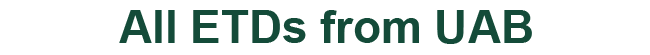### Sturmian Theorems, Asymptotic Behavior for Eigenvalues, and Spectral Theory for Ordinary Differential Equations With Distributional Coefficients

Rudi Weikard

Boris Kunin

Kabe Moen

Ilias Perakis

Gunter Stolz

Dissertation

2022

#### Degree Name by School

Doctor of Philosophy (PhD) College of Arts and Sciences

#### Abstract

We study the theory of ordinary differential equations whose coefficients are distributions of order 0. In the first chapter of the thesis, we present some of the basic facts about the theory of distributions of order 0, the first-order ordinary differential equation with distributional coefficients, and the theory of linear relation associated with these differential equations. In the second chapter, we study the spectral theory of the first order equation with distributional coefficients. In particular, we prove that the adjoint of the minimal relation is equal to the maximal relation, which is a cornerstone for a spectral theory since it ensures the existence and classification of self-adjoint linear relations via the well-known von Neumann theorem about Friedrich extensions. The next chapter of the thesis deals with a collection of Sturm-Liouville type theorems regarding the close connection between the counting number of eigenvalues and the zero set of their associating eigenfunctions. In the last chapter, we establish a result about the existence and asymptotic formula for the eigenvalues of a regular two-point boundary value problem.

COinS